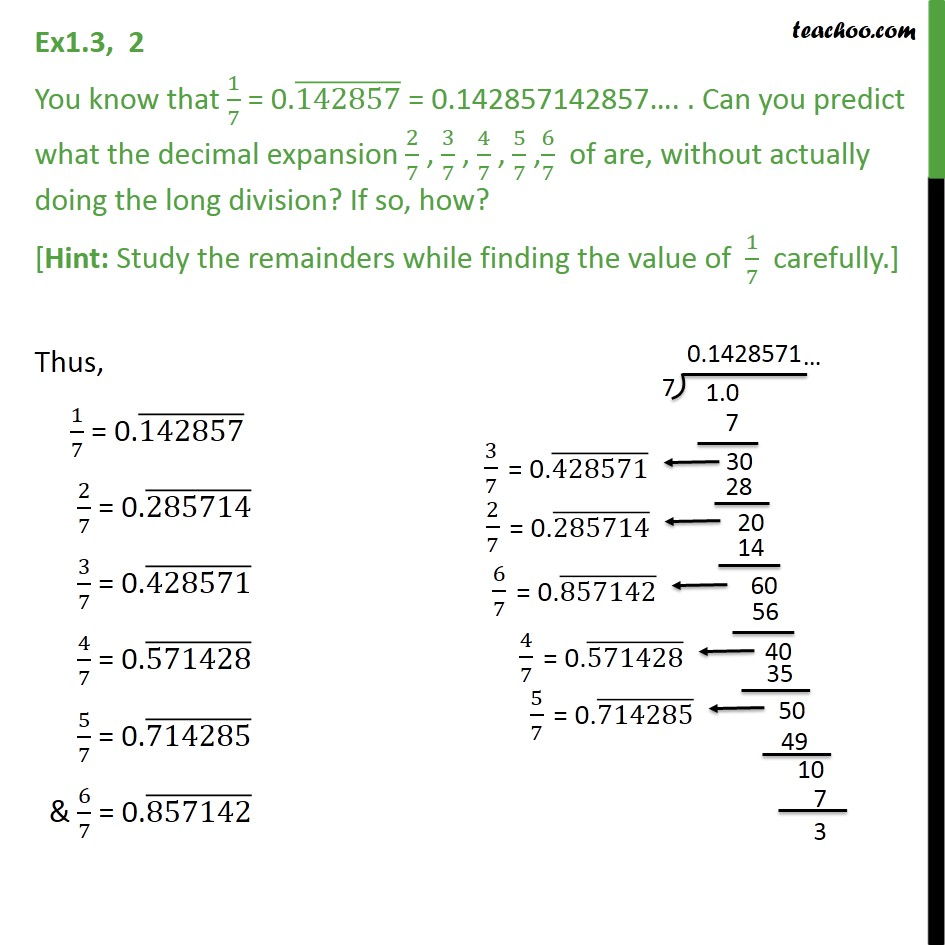1. Chapter 1 Class 9 Number Systems (Term 1)
2. Concept wise
3. Finding decimal expansion

Transcript

Ex1.3, 2 You know that 1/7 = 0.(142857) ̅ = 0.142857142857…. . Can you predict what the decimal expansion 2/7 , 3/7 , 4/7 , 5/7 ,6/7 of are, without actually doing the long division? If so, how? [Hint: Study the remainders while finding the value of 1/7 carefully.] Thus, 1/7 = 0.(142857) ̅ 2/7 = 0.(285714) ̅ 3/7 = 0.(428571) ̅ 4/7 = 0.(571428) ̅ 5/7 = 0.(714285) ̅ & 6/7 = 0.(857142) ̅ .